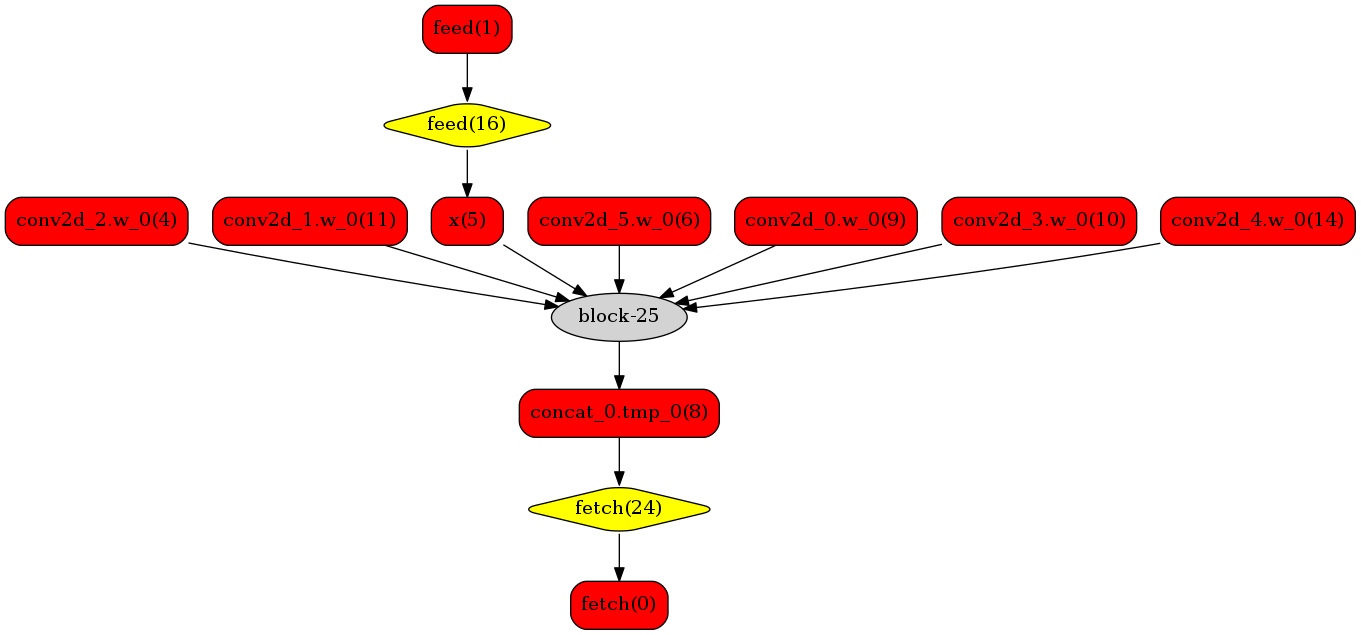# GPU TensorRT 加速推理(NV-GPU/Jetson)¶

## 1. 概要¶

TensorRT 是一个针对 NVIDIA GPU 及 Jetson 系列硬件的高性能机器学习推理 SDK，可以使得深度学习模型在这些硬件上的部署获得更好的性能。Paddle Inference 以子图方式集成了 TensorRT，将可用 TensorRT 加速的算子组成子图供给 TensorRT，以获取 TensorRT 加速的同时，保留 PaddlePaddle 即训即推的能力。在这篇文章中，我们会介绍如何使用 TensorRT 加速推理。

## 2. 环境准备¶

• 电脑上 CUDA、cuDNN、TensorRT 都还没安装的开发者，建议参考 Paddle Inference 提供的预编译包信息，去安装对应版本的CUDA、cuDNN、TensorRT。

• 电脑上已安装 CUDA、cuDNN、TensorRT的开发者，去下载对应版本的 Paddle Inference 预编译包。

Paddle Inference 提供的 Ubuntu/Windows 平台的官方 Release 推理库均支持 TensorRT 加速推理，如果您使用的是以上平台，我们优先推荐您通过以下链接直接下载，或者您也可以参照文档进行源码编译

Note:

1. 从源码编译支持 TensorRT 加速的 Paddle Infenrence 推理库时，你需要设置编译选项 TENSORRT_ROOT 为 TensorRT SDK 的根目录。

2. Windows 支持需要 TensorRT 版本 5.0 以上。

3. 使用 TensorRT 的动态 shape 输入功能要求 TensorRT 的版本在 6.0 以上。

## 3, API 使用介绍¶

• 导入包

• 设置 Config

• 创建 Predictor

• 准备输入

• 执行 Predictor

• 获取输出

```    import numpy as np

def create_predictor():
config.enable_memory_optim()
config.enable_use_gpu(1000, 0)

# 打开TensorRT。此接口的详细介绍请见下文
config.enable_tensorrt_engine(workspace_size = 1 << 30,
max_batch_size = 1,
min_subgraph_size = 3,
use_static = False, use_calib_mode = False)

return predictor

def run(predictor, img):
# 准备输入
input_names = predictor.get_input_names()
for i,  name in enumerate(input_names):
input_tensor = predictor.get_input_handle(name)
input_tensor.reshape(img[i].shape)
input_tensor.copy_from_cpu(img[i].copy())
# 推理
predictor.run()
results = []
# 获取输出
output_names = predictor.get_output_names()
for i, name in enumerate(output_names):
output_tensor = predictor.get_output_handle(name)
output_data = output_tensor.copy_to_cpu()
results.append(output_data)
return results

if __name__ == '__main__':
pred = create_predictor()
img = np.ones((1, 3, 224, 224)).astype(np.float32)
result = run(pred, [img])
print ("class index: ", np.argmax(result))
```

```    config.enable_tensorrt_engine(workspace_size = 1 << 30,
max_batch_size = 1,
min_subgraph_size = 3,
use_static = False, use_calib_mode = False)
```

• workspace_size，类型：int，默认值为1 << 30 （1G）。指定 TensorRT 使用的工作空间大小，TensorRT 会在该大小限制下筛选最优的 kernel 进行推理。

• max_batch_size，类型：int，默认值为1。需要提前设置最大的batch大小，运行时batch大小不得超过此限定值。

• min_subgraph_size，类型：int，默认值为3。Paddle Inference 是以以子图的形式接入 TensorRT 的，为了避免性能损失，当子图内部节点个数大于 min_subgraph_size 的时候，才会将此子图接入 TensorRT 运行。

• precision_mode，类型：paddle_infer.PrecisionType， 默认值为 paddle_infer.PrecisionType.Float32。指定使用 TensorRT 的精度，支持FP32（Float32），FP16（Half），Int8（Int8）。若需要使用 TensorRT int8 离线量化校准，需设定 precision 为 paddle_infer.PrecisionType.Int8 , 且设置 use_calib_mode 为 True。

• use_static，类型：bool， 默认值为 False。如果指定为 True，在初次运行程序的时候会将 TensorRT 的优化信息进行序列化到磁盘上，下次运行时直接加载优化的序列化信息而不需要重新生成。

• use_calib_mode，类型：bool， 默认值为 False。若要运行 int8 离线量化校准，需要将此选项设置为 True。

```// python API
config.enable_tensorrt_dla(0)
// C++ API
config.EnableTensorRtDLA(0);
```

DLA 上对运行的模型有一定要求，详情请可参考链接。当 DLA 遇到模型中的某些不支持的层时，会回退到 GPU 进行推理，目前 DLA 仅支持fp16 和 int8 精度。

## 4. 运行 Dynamic shape¶

```	config.enable_tensorrt_engine(
workspace_size = 1<<30,
max_batch_size=1, min_subgraph_size=5,
use_static=False, use_calib_mode=False)

min_input_shape = {"image":[1,3, 10, 10]}
max_input_shape = {"image":[1,3, 224, 224]}
opt_input_shape = {"image":[1,3, 100, 100]}

config.set_trt_dynamic_shape_info(min_input_shape, max_input_shape, opt_input_shape)
```

Paddle Inference 还提供了另外一份使用动态 shape 方法，此接口不用明确指出输入 shape 范围，但需要准备一些数据来运行模型，以便于收集模型中 Tensor 的大小，使用接口如下：

```    if args.tune:
config.collect_shape_range_info(shape_file)
if args.use_gpu:
config.enable_use_gpu(1000, 0)
if args.use_trt:
# using dynamic shpae mode, the max_batch_size will be ignored.
config.enable_tensorrt_engine(
workspace_size=1 << 30,
max_batch_size=1,
min_subgraph_size=5,
precision_mode=PrecisionType.Float32,
use_static=False,
use_calib_mode=False)
if args.tuned_dynamic_shape:
config.enable_tuned_tensorrt_dynamic_shape(shape_file, True)
```

• Python 样例请访问此处链接

• C++ 样例地址请访问此处链接

## 5. Paddle Inference 适配 TensorRT 原理介绍¶

Paddle Inference 采用子图的形式对 TensorRT 进行集成，当模型加载后，神经网络可以表示为由变量和运算节点组成的计算图。Paddle Inference 对整个图进行扫描，发现图中可以使用 TensorRT 优化的子图，并使用 TensorRT 节点替换它们。在模型的推断期间，如果遇到 TensorRT 节点，Paddle Inference 会调用 TensorRT 库对该节点进行优化，其他的节点调用 Paddle Infenrence 的 GPU 原生实现。TensorRT 在推断期间能够进行 Op 的横向和纵向融合，过滤掉冗余的 Op，并对特定平台下的特定的 Op 选择合适的 Kernel等进行优化，能够加快模型的推理速度。